# Nanoelectronics

Moore's Law and the Future of Solid-State Electronics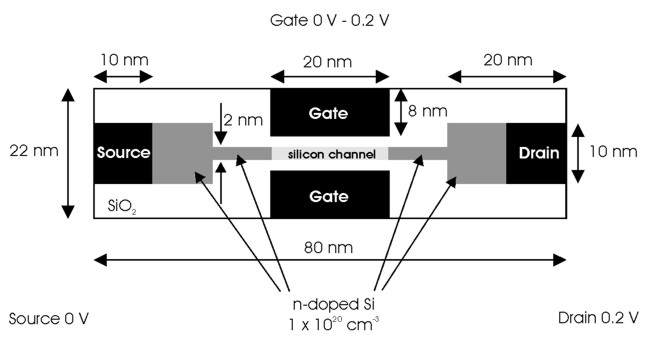## Introduction

As silicon transistors are getting smaller and smaller, eventually quantum mechanical effects will dominate.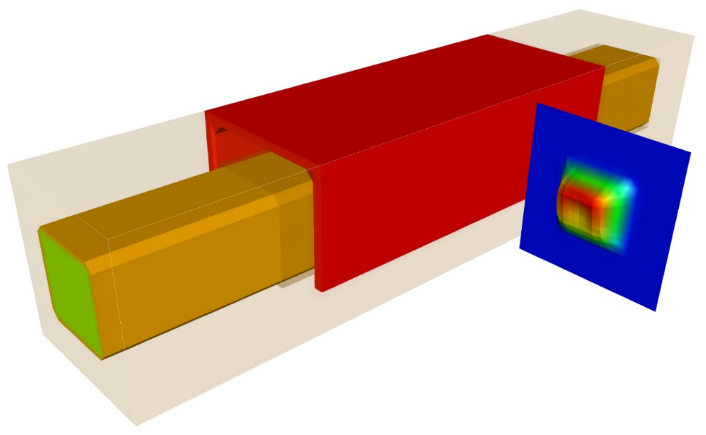## Tutorials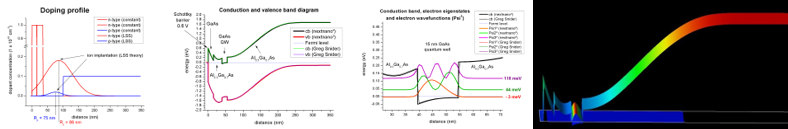## Schrödinger-Poisson

What you can learn:
• heterostructure, doping, 2DEG, AlGaAs/GaAs, user-defined function for the doping profile
• self-consistent Schrödinger-Poisson equation

## HEMTInGaAs/AlInAs HEMT structure (High Electron Mobility Transistor)

What you can learn:
• heterostructure, doping, 2DEG, InGaAs/AlInAs
• strain equation, self-consistent Schrödinger-Poisson equation

## Inverted HEMT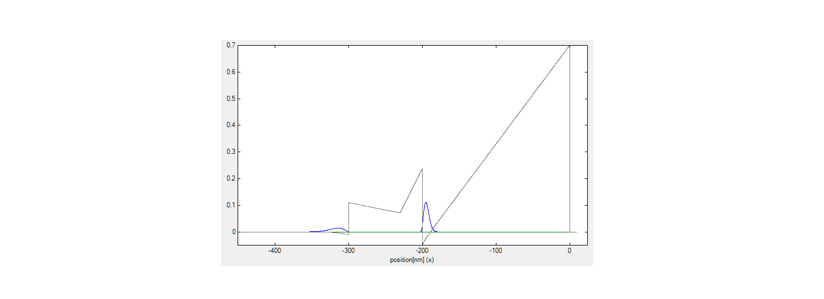AlGaAs/GaAs inverted HEMT structure (High Electron Mobility Transistor) - no online documentation available

What you can learn:
• heterostructure, doping, 2DEG, AlGaAs/GaAs, variables in input file
• self-consistent Schrödinger-Poisson equation

## Si/SiGe MODQW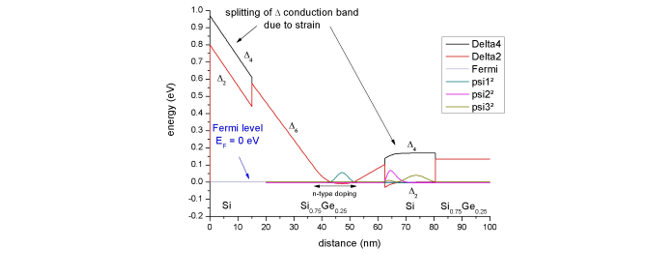Si/SiGe MODQW (Modulation Doped Quantum Well)

What you can learn:
• heterostructure, doping, 2DEG, Si/SiGe, strain, Delta band edge
• strain equation, self-consistent Schrödinger-Poisson equation

## Capacitance-Voltage (MIS)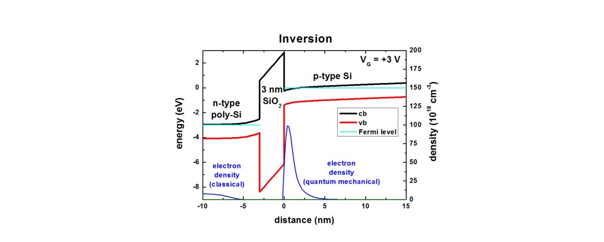What you can learn:
• apply a bias
• self-consistent Schrödinger-Poisson equation

## AlGaN/GaN FET- Two-dimensional electron gas in an AlGaN/GaN field effect transistor (FET)
(nextnano++ tutorial)
- Two-dimensional electron gas in an AlGaN/GaN field effect transistor (FET)
(nextnano³ tutorial)

What you can learn:
• wurtzite, strain, piezoelectricity, pyroelectricity, electron energy levels and wave functions, 2DEG, variables in input file
• strain equation, self-consistent Schrödinger-Poisson equation

## Ultrathin-body Double Gate FET - DG MOSFET (Double Gate Metal Oxide Semiconductor Field Effect Transistor)Double Gate MOSFET (Metal Oxide Semiconductor Field Effect Transistor)

What you can learn:
• 2D and 3D simulations, drift-diffusion, mobility models
• self-consistent Current-Poisson equation in 2D and 3D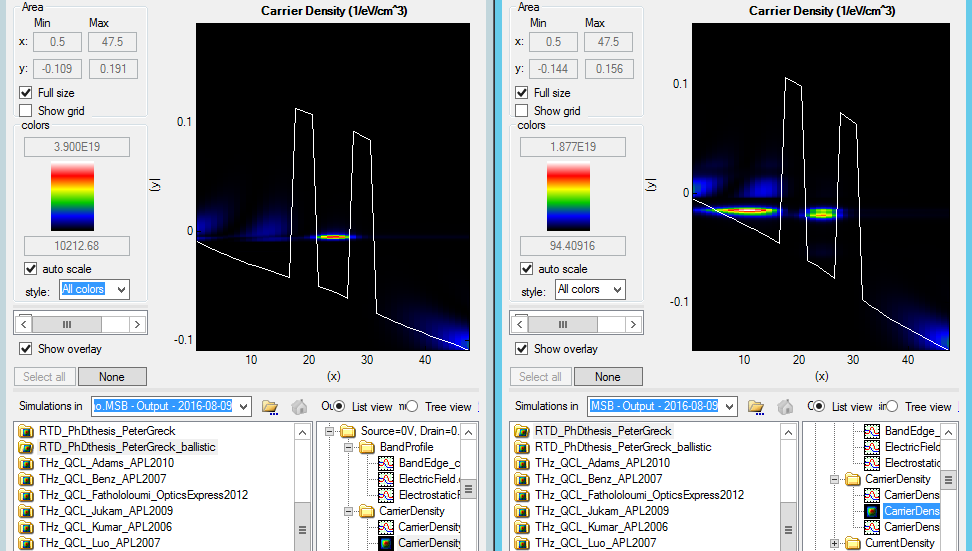## Resonant Tunneling Diode

RTD
Resonant Tunneling Diode - nextnano.MSB software
(Resonant Tunneling Diode - nextnano3 software)
I-V characteristics calculated with a nonequilibrium Green's function approach (NEGF)